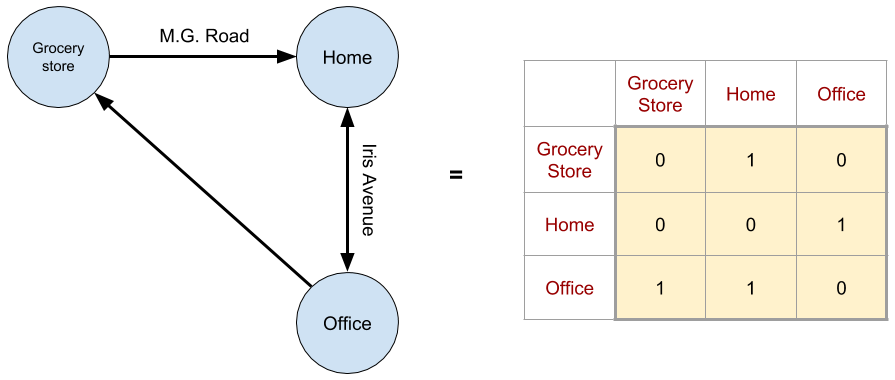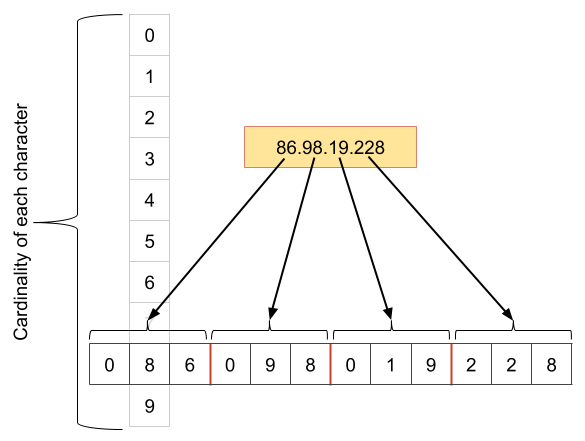# Encoding fixed length high cardinality non-numeric columns for a ML algorithmFigure 1: An simple road network represented as a Graph, where points of interest are nodes and roads connecting them are edges is shown on the left. The corresponding adjacency matrix is shown on the right
`from sklearn.cluster import KMeansimport numpy as npX = np.array([    [0, 1, 0],     [0, 0, 1],     [1, 1, 0]])kmeans = KMeans(n_clusters=2, random_state=0).fit(X)`

# Character EncodingsFigure 4: Expanding IPv4 Address to fixed 12 characters long by padding each section of it with zero(s). Also showing the cardinality of each character which is at most 10
`def transform_ip(ip):    """    If IPv4, equalizes each group and left zero pads to match IPv6 length    If IPv6, converts all to lower case    """    IPV6_LENGTH = 39    IPV4_GROUP_LENGTH = 3 # each group in IPv4 is of this length    if len(ip) < IPV6_LENGTH:        # IPv4 address        groups = ip.split( "." )        equalize_group_length = "".join( map( lambda group: group.zfill(3), groups ))        left_pad_with_zeros = list( equalize_group_length ).zfill( IPV6_LENGTH )        return left_pad_with_zeros    else:        return list(ip.lower())`
`from sklearn.preprocessing import CategoricalEncoderdef one_hot_ip(df):    """    Converts the ipAddress column of pandas DataFrame df, to one-hot    Also returns the encoder used    """    enc = CategoricalEncoder()    ip_df = df.ipAddress.apply( lambda ip: transform_ip(ip) ).apply( pd.Series ) # creates separate columns for each char in IP    X_ip = enc.fit_transform( ip_df ).toarray()    return X_ip, enc`
`pip install git+git://github.com/scikit-learn/scikit-learn.git`